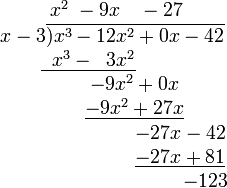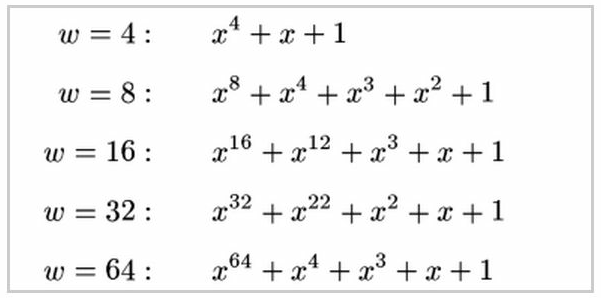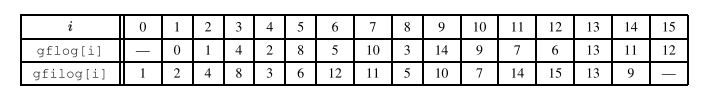# kk Blog —— 通用基础

## date [-d @int|str] [+%s|"+%F %T"]netstat -ltunp## 伽罗华域（Galois Field）上的四则运算

https://blog.csdn.net/shelldon/article/details/54729687

Évariste Galois ，伽罗华（也译作伽瓦罗），法国数学家，群论的创立者。用群论彻底解决了根式求解代数方程的问题，而且由此发展了一整套关于群和域的理论。 本文介绍伽罗华域，以及在伽罗华域上的四则运算方式。伽罗华域上的四则运算实际上是多项式计算，后文中详细介绍。

### 一、相关数学概念

#### 3、单位元

Identity Element，也叫幺元（么元），通常使用e来表示单位元。单位元和其他元素结合时，并不会改变那些元素。 对于二元运算，若ae=a，e称为右单位元；若ea=a，e称为左单位元，若ae=e*a=a，则e称为单位元。

### 二、多项式运算

#### 3、多项式除法#### 4、GF（2w）上的多项式运算

GF（2w）的多项式减法等于加法，例如x ^4 – x4就等于x4 + x4

### 三、伽罗华域

#### 2、有限域GF(2w)：

GF(p)中p必须是一个素数，才能保证集合中的所有元素都有加法和乘法逆元(0除外)。但实际应用中，很多场合需要 0到255这256个数字能组成一个域。但256不是素数，小于256的最大素数为251，如果直接把大于等于251的数截断为250，则会丢失一部分数据。

### 四、本原多项式

GF(23)上有不只一个本原多项式，选一个本原多项式x3+x+1，这8个多项式进行四则运算后 mod (x3+x+1)的结果都是8个之中的某一个。因此这8个多项式构成一个有限域。#### 通过本原多项式生成元素

1、给定一个初始集合，包含0,1和元素x，即 {0,1,x}；
2、将这个集合中的最后一个元素，即x，乘以x，如果结果的度大于等于w，则将结果mod P(x)后加入集合；
3、直到集合有2w个元素，此时最后一个元素乘以x再mod P(x)的值等于1。

 生成元素 多项式表示 二进制表示 数值表示 推导过程 0 0 0000 0 x^0 x^0 0001 1 x^1 x^1 0010 2 x^2 x^2 0100 4 x^3 x^3 1000 8 x^4 x+1 0011 3 x^3*x = x^4 mod P(x) = x+1 x^5 x^2+x 0110 6 x^4*x = (x+1)*x = x^2+x x^6 x^3+x^2 1100 12 x^7 x^3+x+1 1011 11 x^6*x = (x^3+x^2)*x = x^4 +x^3 mod P(x) = x^3+x+1 x^8 x^2+1 0101 5 x^9 x^3+x 1010 10 x^10 x^2+x+1 0111 7 x^9*x=(x^3+x)*x = x^4+x^2 mod P(x) = x^2+x+1 x^11 x^3+x^2+x 1110 14 x^12 x^3+x^2+x+1 1111 15 x^11*x=(x^3+x^2+x)*x = x^4+x^3+x^2 mod P(x) = x^3+x^2+x+1 x^13 x^3+x^2+1 1101 13 x^12*x=(x^3+x^2+1 )*x = x^4+x^3+x mod P(x)= x^3+1 x^14 x^3+1 1001 9 x^13*x=(x^3+x^2+1)*x = x^4+x^3+x mod P(x) = x^3+1 x^15 1 0001 1 x^14*x = (x^3+1)*x = x^4+x mod P(x) = 1

### 五、伽罗华域上的运算

#### 乘法和除法：

1）a7 == 0，此时结果是一个小于指数小于8的多项式，不需要取模计算。

2）a7 == 1，则将x8替换为x4 + x3 + x2 +1，而不用进行除法取模计算，结果为：

xf(x) = (x4 + x3 + x2 +1) + a6x7 + a5x6 + a4x5 + a3x4 + a2x3 + a1x1 + a0x

### 六、查表的原理

GF(2w)是一个有限域，就是元素个数是有限的，但指数k是可以无穷的。所以必然存在循环。这个循环的周期是2w-1（g不能生成多项式 0）。所以当k大于等于2w-1时，gk =gk%(2w-1)#### 查表进行乘法和除法运算的例子

7 * 9 = gfilog[gflog + gflog] = gfilog[10 + 14] = gfilog[24 mod 15] = gfilog = 10

13 / 11 = gfilog[gflog - gflog] = gfilog[13 - 7] = gfilog = 12

### 五、生成GF（2w）gflog表和gfilog表的python代码

#### 参考